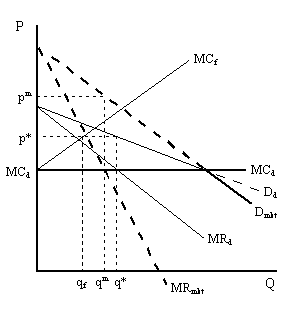Barry Haworth
University of Louisville
Department of Economics
Economics 442

### Strategic Entry Barriers:

The Dominant Firm and Limit Pricing

In some highly concentrated industries, a single ("dominant") firm serves a majority of the market and a group of smaller ("fringe") firms supply the rest. Martin (1994) summarizes the difference between a monopolist and a dominant firm as follows:

"A dominant firm differs from a monopolist in one important respect. The only constraint on the monopolist's behavior is the market demand curve: if the monopolist raises price, some customers will leave the market. Like the monopolist, the dominant firm is large enough to recognize that a price increase will drive some customers from the market. But the dominant firm faces a problem that the monopolist does not: the possibility that a price increase will induce some customers to begin to buy from firms in the fringe of small competitors. That dominant firm, in other words, must take into account the reaction of its fringe competitors."

To best understand how the dominant firm may attempt to prevent entry by strategically setting its price, we need to examine how the dominant firm and its competitive fringe determine output. In the graph (below), we derive the residual demand curve - facing the dominant firm (Dd) - from the MC, or supply curve, of the fringe (MCf) and market demand (Dmkt). That is, we find the "new" demand curve faced by the dominant firm when the market is shared with a competitive fringe. As a monopolist, the dominant firm would charge pm and produce qm.With entry by the fringe, the dominant firm now faces a residual demand curve, rather than the market demand curve. Notice that if the market price falls below the point where the residual demand curve, Dd, crosses the market demand curve, Dmkt, the dominant firm is (once again) a monopolist. This point corresponds with where MCf crosses the vertical axis (where the fringe produces zero output).

Since the dominant firm chooses to produce where current profits are maximized (i.e. where MRd = MCd) the price falls to p* and output responds by rising to q*. The competitive fringe induces the dominant firm to exercise some restraint in price setting. As long as this restraint is present, the market price will remain lower. At the price p*, consumers will be willing to buy more than q* units and so we find the fringe producing as well. The fringe firm(s) will produce at qf (where p* = MCf).

As the dominant firm confronts the entry of the fringe, what is the proper course of action? One answer concerns the strategic use of pricing. There are several approaches: static limit pricing and dynamic limit pricing. Let's examine these individually.

Static Limit pricing. One option is that the firm sets a price that prevents the fringe from entering the market (i.e. causes qf = 0). The dominant firm could do this by lowering the price - from pm to MCd - causing the dominant firm's economic profits to fall to zero. One problem with static limit pricing concerns whether a rational firm would ever engage in non-profit maximizing behavior. That is, the firm could make higher (current) profits by setting a different price. Naturally, the dominant firm is concerned with future profits as well, and so the answer depends upon both current and future profits.

Dynamic Limit pricing. Another option is one where the firm considers what is called the present discounted value of the stream of profits it receives over time. There are two ways of viewing this approach. Each way concerns how the firm is able to "gaze into the future." Suppose the firm has myopic foresight, and can only view each period as it occurs. In this event, they will take entry as given and maximize current profits. In the graph above, this leads to the price p*. As entry continues to expand the size of the fringe, the fringe "supply" curve becomes flatter. A flatter fringe supply curve causes a flatter residual demand curve for the dominant firm. Thus, the dominant firm's price will fall as the fringe expands.

If the dominant firm has perfect foresight, then they will maximize the present discounted value of profits. Likewise, the firm takes entry as given and allows the fringe to expand over time. Essentially, the difference is that the myopic foresight price will exceed the perfect foresight price. In both cases, however, the price falls over each successive period until reaching the limit price (here, note that the limit price is MCd - the perfectly competitive price).

Either way, whether there is perfect or myopic foresight, the dominant firm reacts passively to the expansion of the fringe. The dominant firm seeks only to maximize their profits in some sense (either the present discounted value or current value per period). As a result, this model becomes a way of predicting movements in price and market share for industries like the auto or steel industry where a dominant firm or set of firms face competition from smaller entrants.